Next: High Momentum Limit Up: Vertex Fitting Previous: Vertex Fitting with Error

## Inclusion of Geometrical Constraints

In the last subsection, we tried to determine the common vertex of given ntrack tracks without touching the track parameter vectors themselves: these helical tracks do not necessarily pass through the common vertex. Here, we require these tracks to originate from a common vertex: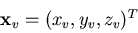so that i-th track, for instance, can be parametrized as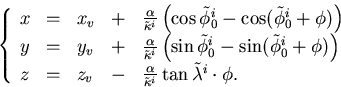(38)
Notice that, for a given trial vertex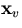, the track parameter vector has now only three components: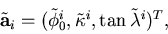since the track has to pass through the pivotand therefore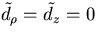.

In order to calculate the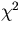, we need to transform the pivot of the i-th track from the trial vertexto the pivot of the corresponding measured track. This induces the following change of the helix parameters: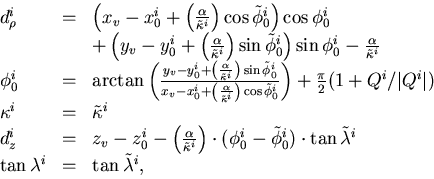(39)
which gives the helix parameter vector: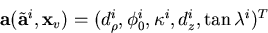to be compared with the corresponding measured track parameter vector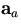.Thus, we arrive at thedefinition: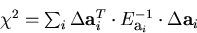(40)
with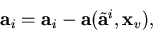the minimization of which determinies the parameter vector: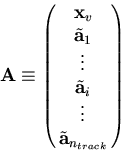containing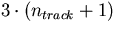components. Notice that the necessary calculations of the first and the second derivatives of therequire only the evaluation of the transformation matrix: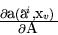and what then follows is the same as with the last subsection.Next: High Momentum Limit Up: Vertex Fitting Previous: Vertex Fitting with Error
Keisuke Fujii
12/4/1998